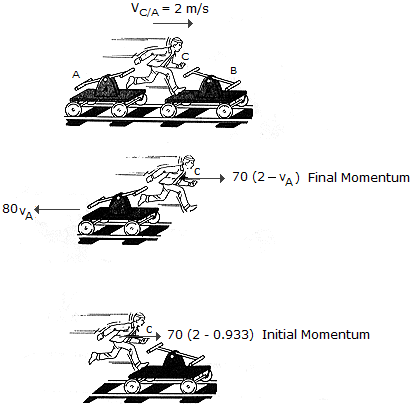Engineering Mechanics - KOP: Impulse and Momentum - Discussion

Discussion :: KOP: Impulse and Momentum - General Questions (Q.No.10)

10.The two handcars A and B each have a mass of 80 kg. Both cars are initially at rest. Both cars start from rest before the man jumps. If the man C has a mass of 70 kg and jumps from A with a horizontal relative velocity of vC/A = 2 m/s and lands on B, determine the velocity of each car after the jump. Neglect the effects of rolling resistance.

 [A]. vA = 0.933 m/s vB = 0.498 m/s [B]. vA = 1.750 m/s vB = 0.933 m/s [C]. vA = 0.498 m/s vB = 1.750 m/s [D]. vA = 1.750 m/s vB = 1.750 m/s

Explanation:

No answer description available for this question.

 Alexander said: (Jan 13, 2022) Ma = 80 kg Mb = 80kg Mc = 70 kg. Va1 = 0m/s Vb1 = 0 m/s Vc = 2m/s. Va2 = ? Vb 2 = ?. Vb2 ==> Mb * Vb + Mc * Vc = Mb+c * Vb2. 80kg *0 + 70kg*2m/s = 150kg * Vb2. Vb2 = 140/150. Vb2 = 0.9333 m/s. Va2 ==> 80*0.93 ==> 74.4. 70* (2-0.93) ==> 74.9. Va2 = 74.9-74.4 ==> 0.5m/s.